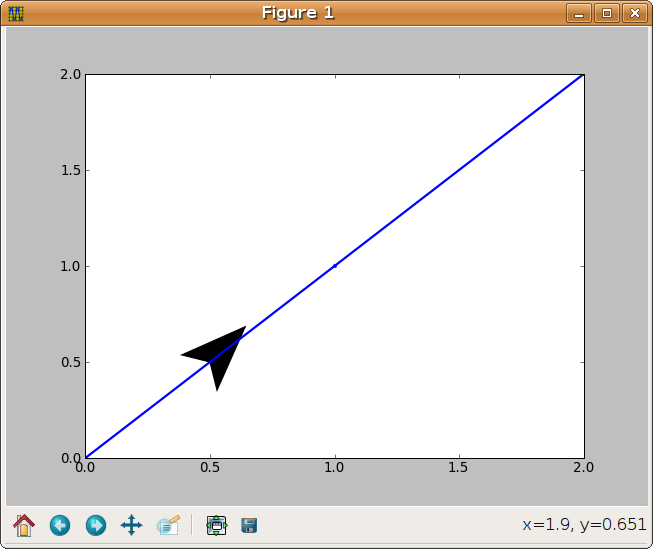# add an arrow to a lineEnd

Hello,

the original problem that I have is to add an arrow to the end of a
plotted line. I tried this

from pylab import *
x=linspace(0, 1.85, 100)
y=sin(x)

dx=x[-1] - x[-2]
dy=y[-1] - y[-2]
plot(x,y,lw=2)
arrow(x[-2], y[-2], dx, dy,
width=.02,
lw=0, overhang=.1)

But this is ugly. And when I zoom in the arrowsize isnt scaled with the
linewidth.

Any idea instead to switch to the pyx package?

By,

Friedrich

Hello,

I want do add an arrow *tip* to a line with the same angle.

I have tried this:

from pylab import *
from matplotlib import collections, transforms

def MyArrow(ax, x, y, ang, scale=50, over=.5, color='k'):
ar = [(0,0), (-.5,-over), (0,1), (.5,-over), (0,0)]
col = collections.PolyCollection(
[ar],
offsets=(x, y),
transOffset=ax.transData,
color=color
)
col.set_transform(transforms.Affine2D().rotate(ang).scale(scale))

plot([0,1,2], '.-', lw=2)
MyArrow(gca(), .5, .5, -pi/4)
draw()

and I get an arrow tip without the same line angleSee the attached
image.

Can you help me to get the right transformation?

Thanks,

FriedrichIt should behave like a marker: it should not resize when I zoom in.

By, Friedrich

···

On Tue, Jun 03, 2008 at 03:55:48PM +0200, Friedrich Hagedorn wrote:

I want do add an arrow *tip* to a line with the same angle.